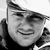Announcements
Applications are open for the 2024 Qlik Luminary Program. Apply by December 15 here.
cancel
Showing results for
Did you mean:Partner - Contributor III

## Solution to Change calculation per field selection

Hi everyone,

Seeking guidance and help on the best performance solution.

Problem: Using a filter panel to change the calculation on charts

Imagine that I need to have a [field] (not available in the DataModel of the Application) with the following values:

• Interaction
• InteractionAggr
• InteractionAvg

This field needs to be available in a Filter Panel visualization. Once the user switch between different values, the charts need to update their calculations.

My idea would be to have a general calculation that is going to change the measure taking into account the [Field] selection, for example:

Sum( {<Set Analysis>} [\$(vMeasureToChange)] )

Then, depending on the user selection, the calculation would be as the following:

• [field] = 'Interaction'
• Sum( {<Set Analysis>} [Interaction] )
• [field] = 'InteractionAggr'
• Sum( {<Set Analysis>} [InteractionAggr] )
• [field] = 'InteractionAvg'
• Sum( {<Set Analysis>} [InteractionAvg] )

In this way, i would avoid performance issues with If's and picks in the front-end.

However, i need guidance on how to do this.

Is it possible to work only in the front-end + variables? This would be the perfect scenario taking into account Environment limitations that we have at this moment. Tried to use valuelist() function in the Filter Panel visualization, but it does not allow any value to be selected in the DataModel, as long as it is a synthetic dimension.

Do i need to work in the Script in order to make this behaviour work? If so, what's the guidance?

Thank you for the help and guidance.

Best regards.

1 Solution

Accepted SolutionsPartner - Contributor III
Author

Hey everyone,

Seems that i found a solution. Don't know if it would be the best in terms of performance, but here it is:

1. Create a Island Table with the values that we need to provide for the user selections

Island_table:
[
Interaction Type
Interaction
InteractionAggr
InteractionAvg
];

2. Create a variable that looks at the selection done by the user

\$(vTeste1)

=if(GetFieldSelections([Interaction Type])='Interaction', 'Interaction',
if(GetFieldSelections([Interaction Type])='InteractionAggr', 'InteractionAggr', 'InteractionAvg'))

3.Have a general calculation

Sum( [\$(vTeste1)] )

Have a nice weekend!

2 RepliesMVP

You could try this:

Sum( {<Set Analysis>} [\$(=(Maxstring(field)))] )Partner - Contributor III
Author

Hey everyone,

Seems that i found a solution. Don't know if it would be the best in terms of performance, but here it is:

1. Create a Island Table with the values that we need to provide for the user selections

Island_table:
[
Interaction Type
Interaction
InteractionAggr
InteractionAvg
];

2. Create a variable that looks at the selection done by the user

\$(vTeste1)

=if(GetFieldSelections([Interaction Type])='Interaction', 'Interaction',
if(GetFieldSelections([Interaction Type])='InteractionAggr', 'InteractionAggr', 'InteractionAvg'))

3.Have a general calculation

Sum( [\$(vTeste1)] )

Have a nice weekend!Tags
Community Browser